# Texas Go Math Grade 2 Lesson 2.4 Answer Key Locate Numbers on a Number Line

Refer to our Texas Go Math Grade 2 Answer Key Pdf to score good marks in the exams. Test yourself by practicing the problems from Texas Go Math Grade 2 Lesson 2.4 Answer Key Locate Numbers on a Number Line.

## Texas Go Math Grade 2 Lesson 2.4 Answer Key Locate Numbers on a Number Line

Explore

Draw points on the number lines for the problem.
Ellie’s number lineAlfredo’s number line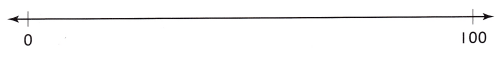FOR THE TEACHER • Read the problem to children. Ellie and Alfredo each want to draw points for 28 and 63 on their number lines. Where should the points be? Draw and label the points.
Ellie number line: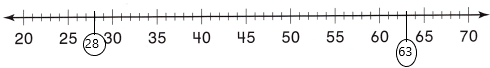Alfredo number line: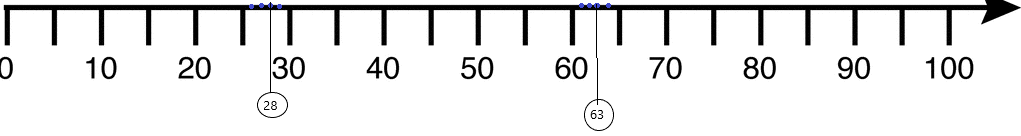Explanation:
– A number line is a pictorial representation of numbers on a straight line. It’s a reference for comparing and ordering numbers. It can be used to represent any real number that includes every whole number and natural number. Just to recollect, the whole number is a set of numbers that include all counting numbers (1, 2, 3,4,5,6 …….) and zero (0), whereas the natural number is the set of all counting numbers i.e. 1, 2, 3, 4, 5, 6……..
– Writing numbers on a number line make it easier to compare the numbers. From the above figure, we can see that the integers were placed. In that, we have to point out the 28 and 63.
– Check the number line given and how it was divided.
– In the above-given number line we can count by ones and point out the 28 and 63 easily.
– Map the points and highlighted with circle.

Math Talk

Mathematical Processes

Describe how you decided where the points should go on Alfredo’s number line.
Answer: I divided it by 5 parts up to the number 100.
Explanation:
1. Every point of a number line is assumed to correspond to a real number and every real number to a point.
2. The integers are often shown as specially-marked points evenly spaced on the line. Although this image only shows the integers from 0 to 100, the line includes all real numbers, continuing forever in each direction, and also numbers that are between the integers.
3. On the number line, the distance between two points is the unit length if and only if the difference of the represented numbers equals 1. Other choices are possible. It could be equals to 5, 10, and so on…
4. Likewise I divided the intervals and marked the dots.
5. Mapped the numbers with the help of dots and highlighted them with the circle.Model and Draw

This number line shows counting by tens. What numbers belong at the points?Explanation:
1. Here is a number line between 240 and 340.
2. There are 10 lines dividing the total distance into 10 equal parts.
3. One whole is divided into 10 equal parts.
4. At each new line we are adding 1.
5. The digit after the point increase by 1 each time.
6. Then we have 240, 241, 242, 243, 244, 245, 246, 247, 248, 249, 250, and so on… continues up to 350.
7. Here we counting by 10’s it means we need to add 10 to the 240
240+10=250
250+10=260 and so on…
Draw and label points for the numbers 350 and 500 on this number line.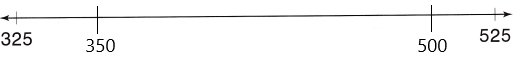Explanation:
1. The above-given numbers we need to plot 350 and 500
2. The number line has given between 325 and 525.
3. Here I skip count by 25’s to plot the answer.
4. adding 25 to the 325 then we get:
325+25=350
350+25=375
375+25=400
400+25=425
425+25=450
450+25=475
475+25=500
500+25=525.
But the number line didn’t mark all the numbers.
5. Close to the 325, 350 was marked and close to the 525, 500 were marked.
Share and Show

Question 1.
Write numbers for the points on the number line.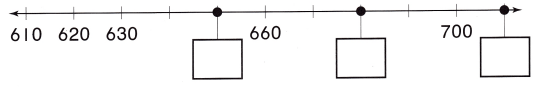1. Here is a number line between 610 and 710.
2. There are 10 lines dividing the total distance into 10 equal parts.
3. One whole is divided into 10 equal parts.
4. At each new line we are adding 1.
5. The digit after the point increase by 1 each time.
6. Then we have 610, 611, 612, 613, 614, 615, 616, 617, 618, 619, 620 and so on… continues up to 710.
7. Here we counting by 10’s it means we need to add 10 to the 610 then we get: 610+10=610
610+10=620
620+10=630
630+10=640
640+10=650 and so on…
8. Now write the given boxes by adding 10 to every number each time.

Question 2.
Draw and label points for 137 and 240 on the number line.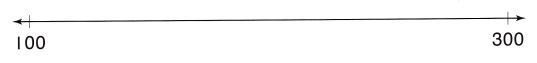Explanation:
1. Here is a number line between 100 and 300.
2. Here I count by ’40s means adding 40 to the 100 and continuing adding to the next number.
3. 100+40=140
140+40=180
180+40=220
220+40=260
260+40=300
3. The above-given numbers need to plot on the number line. those are 137 and 240
4. I represented the 100, 140, 180, 220, 260, 300 on the number line and choose the intervals between the numbers which are closed to 137 and 240. Mapped the points and highlighted them with circles.

Problem Solving

Question 3.
Write numbers for the points on the number line.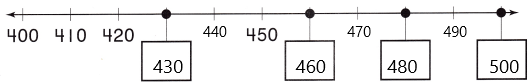Explanation:
1. Here is a number line between 400 and 500
2. There are 10 lines dividing the total distance into 10 equal parts.
3. One whole is divided into 10 equal parts.
4. At each new line we are adding 1.
5. The digit after the point increase by 1 each time.
6. Then we have 410, 411, 412, 413, 414, 415, 416, 417, 418, 419, 420 and so on… continues up to 500.
7. Here we counting by 10’s it means we need to add 10 to the 400 then we get: 400+10=410
410+10=420
420+10=430
430+10=440
440+10=450 and so on…
8. Now write the given boxes by adding 10 to every number each time.

Question 4.
Draw and label points for 220, 258, and 370.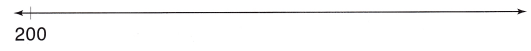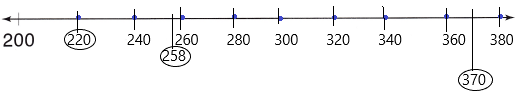Explanation:
1. Here is a number line between 200 and 380.
2. Here I count by ’20s means adding 20 to the 200 and continuing adding to the next number.
3. 200+20=220
220+20=240
240+20=260
260+20=280
280+20=300 and so on.. up to 380.
3. The above-given numbers need to plot on the number line. those are 220, 258 and 370
4. Marked the points on the number line and highlighted them with circles.

Question 5.
H.O.T. Multi-Step One of these points is for the number 200. What numbers might the other points be? Label all the points.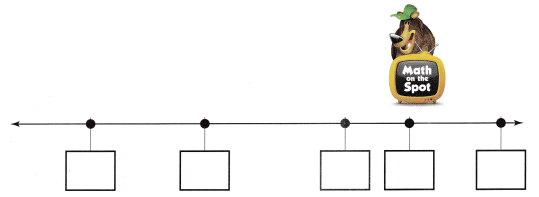Explanation:
1. I choose the first box to be kept at 200.
2. From the 200 I count by 10’s and keep numbers in the boxes.
3. Adding 10 to the 200 we get 210.
4. 200+10=210
210+10=220
220+10=230
230+10=240 and so on…

Question 6.
H.O.T. There are points on this number line for the numbers 430, 275, 360, and a mystery number. Label all points with numbers.Answer: The mystery number is 150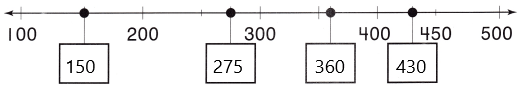Explanation:
1. here the number line starts at 100 and ends at 500
2. For every number added 50 each time.
3. By keenly observing the point it is the centre of 100 and 200. So it was definitely 150.
4. And the remaining numbers are given 430, 275, 360, and the mystery number which is needed to be found out.
5. Therefore, we can conclude that the mystery number is 150.

Question 7.
Record Alex drew this number line. Which is the number for the point on the number line?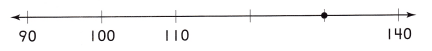(A) 90
(B) 130
(C) 120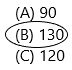Explanation:1. here the number line starts at 90 and ends at 140
2. We count by 10’s each time to the next number.
Thus, we get:
90+10=100
100+10=110
110+10=120
120+10=130
130+10=140
3. The above-given figure has point with a black dot which we need to write the belonged number to that point.
4. Therefore, continuing to add 10 to the next number. Hence the belonging number is 130.

Question 8.
Representations Sandy drew this number line. Which is the number for the point on the number line?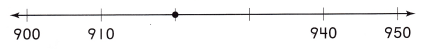(A) 930
(B) 950
(C) 920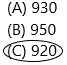Explanation:1. here the number line starts at 900 and ends at 950
2. We count by 10’s each time to the next number.
Thus, we get:
900+10=910
910+10=920
920+10=930
930+10=940
940+10=950
3. The above-given figure has point with a black dot which we need to write the belonged number to that point.
4. Therefore, continuing to add 10 to the next number. Hence the belonging number is 920.

Question 9.
TEXAS Test Prep Which is the number for the point on the number line?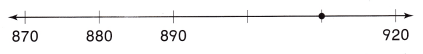(A) 910
(B) 900
(C) 915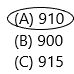Explanation:1. here the number line starts at 870 and ends at 920
2. We count by 10’s each time to the next number.
Thus, we get:
870+10=880
880+10=890
890+10=900
900+10=910
910+10=920
3. The above-given figure has point with a black dot which we need to write the belonged number to that point.
4. Therefore, continuing to add 10 to the next number. Hence the belonging number is 910.

TAKE HOME ACTIVITY • Ask your child to show how he or she solved an exercise in the lesson.

### Texas Go Math Grade 2 Lesson 2.4 Homework and Practice Answer Key

Question 1.
Write numbers for the points on the number line.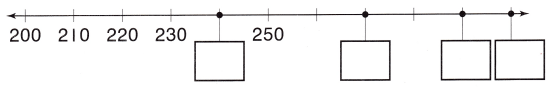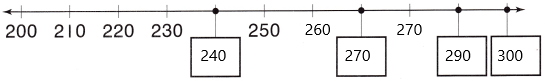Explanation:
1. Here is a number line between 200 and 300
2. There are 10 lines dividing the total distance into 10 equal parts.
3. One whole is divided into 10 equal parts.
4. At each new line we are adding 1.
5. The digit after the point increase by 1 each time.
6. Then we have 210, 211, 212, 213, 214, 215, 216, 217, 218, 219, 220 and so on… continues up to 300.
7. Here we counting by 10’s it means we need to add 10 to the 200 then we get: 200+10=210
210+10=220
220+10=230
230+10=240
240+10=250 and so on…
8. Now write the given boxes by adding 10 to every number each time.

Question 2.
Draw and label points for 310, 348, and 420.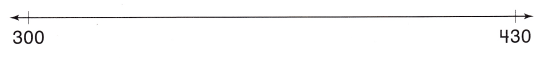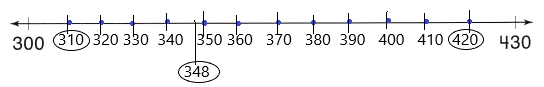Explanation:
1. Here is a number line between 400 and 500
2. There are 10 lines dividing the total distance into 10 equal parts.
3. One whole is divided into 10 equal parts.
4. At each new line we are adding 1.
5. The digit after the point increase by 1 each time.
6. Then we have 310, 311, 312, 313, 314, 315, 316, 317, 318, 319, 320 and so on… continues up to 430.
7. Here we counting by 10’s it means we need to add 10 to the 300 then we get: 300+10=310
310+10=320
320+10=330
330+10=340
340+10=350 and so on…
8. Now write the given boxes by adding 10 to every number each time.
9. Now mark the points and highlight them with circles.

Problem Solving

Solve.

Question 3.
Multi-Step There are points on this number line for the numbers 525, 560, and a mystery number. Label all points with numbers.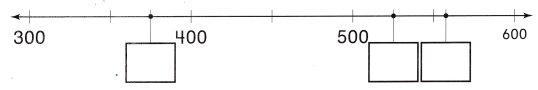Answer: the mystery number is 370Explanation:
1. here the number line starts at 300 and ends at 600
2. For every number added 50 each time.
3. By keenly observing the point it is the centre of 350 and 400. So it was definitely 370.
4. And the remaining numbers are given 525, 560, and the mystery number which is needed to be found out.
5. Therefore, we can conclude that the mystery number is 370.

Lesson Check

Question 4.
Which is the number for the point on the number line?(A) 780
(B) 770
(C) 800Explanation: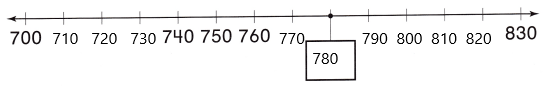1. Here the number line starts at 700 and ends at 830.
2. We count by 10’s each time to the next number.
Thus, we get:
700+10=710
710+10=720
720+10=730
730+10=740
740+10=750
750+10=760
760+10=770
770+10=780 and so on…
3. The above-given figure has point with a black dot which we need to write the belonged number to that point.
4. Therefore, continuing to add 10 to the next number. Hence the belonging number is 780.

Question 5.
Emma drew this number line. Which is the number for the point on the number line?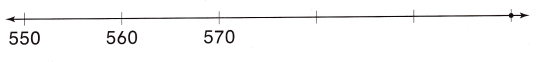(A) 590
(B) 610
(C) 600Explanation: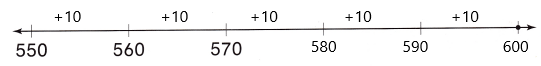1. Here the number line starts at 550 and ends at 600.
2. We count by 10’s each time to the next number.
Thus, we get:
550+10=560
560+10=570
570+10=580
580+10=590
590+10=600
3. The above-given figure has point with a black dot which we need to write the belonged number to that point.
4. Therefore, continuing to add 10 to the next number. Hence the belonging number is 600.

Question 6.
Sandy drew this number line. Which could be the number for the point on the number line?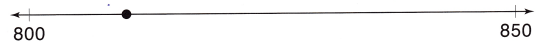(A) 840
(B) 900
(C) 810Explanation:1. Here the number line starts at 800 and ends at 850.
2. We count by 10’s each time to the next number.
Thus, we get:
800+10=810
810+10=820
820+10=830
830+10=840
840+10=850
3. The above-given figure has point with a black dot which we need to write the belonged number to that point.
4. Therefore, continuing to add 10 to the next number. Hence the belonging number is 810.

Scroll to Top